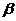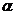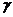Chapter 19, Problem 20QAP### Introductory Chemistry: A Foundati...

9th Edition
Steven S. Zumdahl + 1 other
ISBN: 9781337399425

#### Solutions

Chapter
Section### Introductory Chemistry: A Foundati...

9th Edition
Steven S. Zumdahl + 1 other
ISBN: 9781337399425
Textbook Problem
12 views

# . Which of the following nuclear processes results in a decrease in the neutron-to-proton ratio? Justify your answer. a.particle production b. Positron production c. Electron capture d.particle production e.ray production

Interpretation Introduction

(a)

Interpretation:

If the beta particle production results in decrease in neutron to proton ratio or not should be determined.

Concept Introduction:

The decaying of an atom takes place by three type of decay such as alpha decay, beta decay, gama decay.

In alpha decay, an alpha particle with mass 4m and electron 2e removed from nuclide while in beta decay two type of decay are possible beta (+) and beta (-). In gamma decay, nuclide will remain same to the original one.

Explanation

The beta particle production is shown below:

82X210   83X210+ e-

Neutron /proton ratio of reactant

Interpretation Introduction

(b)

Interpretation:

If the Positron production results in decrease in neutron to proton ratio or not should be determined.

Concept Introduction:

The decaying of an atom takes place by three type of decay such as alpha decay, beta decay, gama decay.

In alpha decay, an alpha particle with mass 4m and electron 2e removed from nuclide while in beta decay two type of decay are possible beta (+) and beta (-). In gamma decay, nuclide will remain same to the original one.

Interpretation Introduction

(c)

Interpretation:

If the Electron capture production results in decrease in neutron to proton ratio or not should be determined.

Concept Introduction:

The decaying of an atom takes place by three type of decay such as alpha decay, beta decay, gama decay.

In alpha decay, an alpha particle with mass 4m and electron 2e removed from nuclide while in beta decay two type of decay are possible beta (+) and beta (-). In gamma decay, nuclide will remain same to the original one.

Interpretation Introduction

(d)

Interpretation:

If the Alpha particle production results in decrease in neutron to proton ratio or not should be determined.

Concept Introduction:

The decaying of an atom takes place by three type of decay such as alpha decay, beta decay, gama decay.

In alpha decay, an alpha particle with mass 4m and electron 2e removed from nuclide while in beta decay two type of decay are possible beta (+) and beta (-). In gamma decay, nuclide will remain same to the original one.

Interpretation Introduction

(e)

Interpretation:

If the Gama ray production results in decrease in neutron to proton ratio or not should be determined.

Concept Introduction:

The decaying of an atom takes place by three type of decay such as alpha decay, beta decay, gama decay.

In alpha decay, an alpha particle with mass 4m and electron 2e removed from nuclide while in beta decay two type of decay are possible beta (+) and beta (-). In gamma decay, nuclide will remain same to the original one.

### Still sussing out bartleby?

Check out a sample textbook solution.

See a sample solution

#### The Solution to Your Study Problems

Bartleby provides explanations to thousands of textbook problems written by our experts, many with advanced degrees!

Get Started